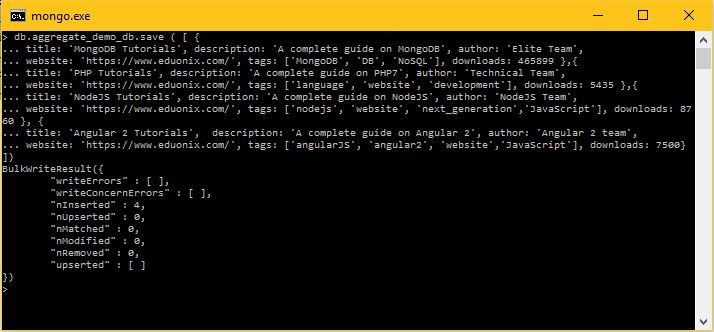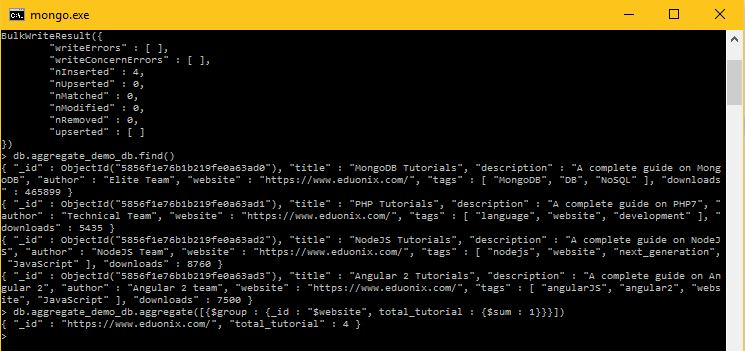# Learn about the Aggregation Method in MongoDB

0
1898In this chapter, we are going to explore the ‘aggregate’ method to operate on the documents present inside a collection of MongoDB. This method collects the records from the collection’s document to process and return a single result such as sum, count, max, min, avg, etc.

Aggregation in MongoDB
If we talk about RDBMS, then aggregate functions are those which process the records based on certain criteria and compute the result such as ‘count (*)’ function to count the number of rows in a table. In the similar manner, the ‘aggregate’ method in MongoDB helps group together the values from multiple documents present inside a collection to perform various operations on the grouped data which returns a single result such as number of occurrence of a record across multiple documents in a collection.

The ensureIndex () Method
In MongoDB, we use the ‘aggregate ()’ method to perform various aggregation operations. The following is the basic syntax for the ‘aggregate ()’ method in MongoDB.

Example
The following is an example on using the ‘aggregate ()’ method. Let’s insert 4 documents into a collection known as ‘aggregate_demo_db’.Explanation of the code

• Here, we are using ‘aggregate_demo_db’ as the collection name of MongoDB.
• Next, we are using the ‘save ()’ method to insert four records together as the bulk write operation in the MongoDB.
• Lastly, we are using the ‘aggregate ([{\$group: {_id: “\$website”, total_tutorial: {\$sum: 1}}}])’ method to calculate the number of occurrences of website URL across all documents present in collection ‘aggregate_demo_db’.
• Since, the website URL occurres 4 times ‘https://www.eduonix.com/’ when we inserted 4 records in the collection. Therefore, the criteria given the aggregate function will return the count as 4 as shown below.Note: – In the above example, we have grouped documents by field website which is present in all the four documents which we inserted in the collection ‘aggregate_demo_db’. If we compare the above operation with that of RDBMS databases like Oracle, DB2, MySQL, etc. then it will be equivalent to the following SQL.

Aggregation Expressions in MongoDB
There are total 8 aggregation expressions available in MongoDB. They are described along with an example as follows.

 S No. Aggregation Expression Description Example 1. \$sum This expression is used to sum up the defined value from all documents present inside a particular collection in MongoDB. db.demo.aggregate ([{\$group : {_id : “\$website”, total_tutorial : {\$sum : “\$downloads”}}}]) 2. \$avg This expression is used to calculate the average of all given values from all the documents present inside a collection. db.demo.aggregate ([{\$group : {_id : “\$website”, total_tutorial : {\$avg : “\$downloads”}}}]) 3. \$min This expression is used to get the minimum of the corresponding values from all documents present inside a collection. db.demo.aggregate([{\$group : {_id : “\$website”, total_tutorial : {\$min : “\$downloads”}}}]) 4. \$max This expression is used to get the maximum of the corresponding values from all documents present inside a collection. db.demo.aggregate([{\$group : {_id : “\$website”, total_tutorial : {\$max : “\$downloads”}}}]) 5. \$push This expression is used to insert the value to an array in the resulting document. db.demo.aggregate([{\$group : {_id : “\$author”, website : {\$push: “\$website”}}}]) 6. \$addToSet This expression is used to insert the value to an array in the resulting document but does not create duplicates. db.demo.aggregate([{\$group : {_id : “\$author “, website : {\$addToSet : “\$website”}}}]) 7. \$first This expression is used to get the first document from the source documents according to the grouping. Usually, this makes sense when it is used together with some previously applied “\$sort”-stage. db.demo.aggregate([{\$group : {_id : “\$author “, first_url : {\$first : “\$website”}}}]) 8. \$last This expression is used to get the last document from the source documents according to the grouping. Usually, this makes sense when it is used together with some previously applied “\$sort”-stage. db.demo.aggregate([{\$group : {_id : “\$author”, last_url : {\$last : “\$website”}}}])

Conclusion:
In this chapter, we have learnt about the practical use of the ‘aggregate ()’ method with the help of multiple examples.